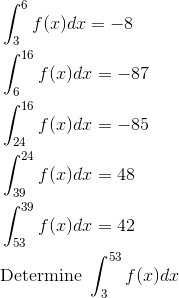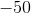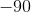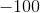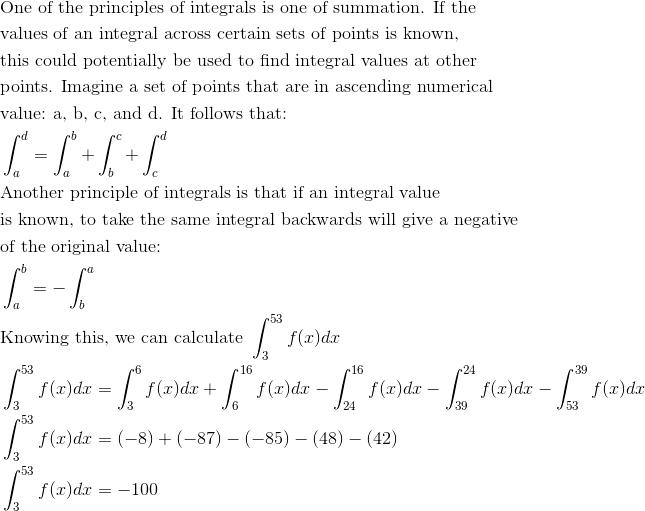# AP Calculus AB : Basic properties of definite integrals (additivity and linearity)

## Example Questions

← Previous 1 3

### Example Question #1 : Basic Properties Of Definite Integrals (Additivity And Linearity)

If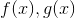are continuous functions,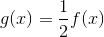,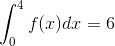, and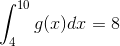, find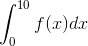.Not enough informationExplanation:

We proceed as follows. (Start)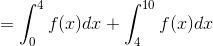. (Break up the integral using the additive rule.)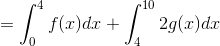. (We don't have information about the 2nd integral, so we solve our first equation forand replace it in this integral.)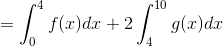. (Factor out theusing linearity.)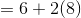. (Substitute in what we were given.)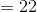.

### Example Question #2 : Basic Properties Of Definite Integrals (Additivity And Linearity)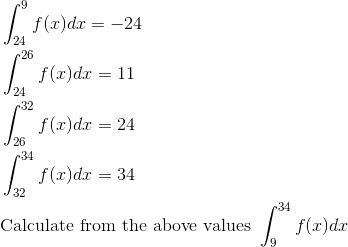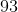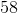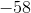Explanation: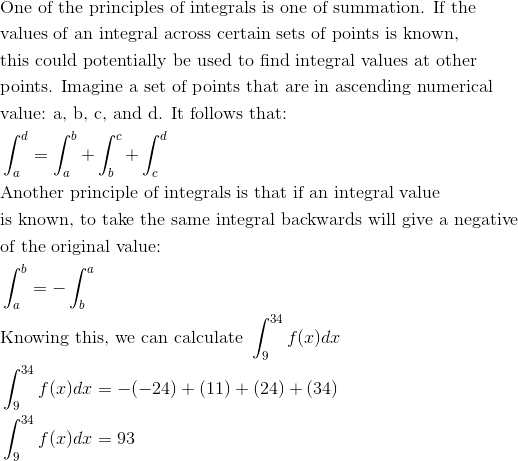### Example Question #3 : Basic Properties Of Definite Integrals (Additivity And Linearity)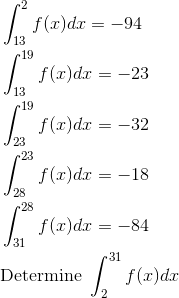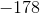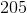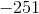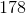Explanation: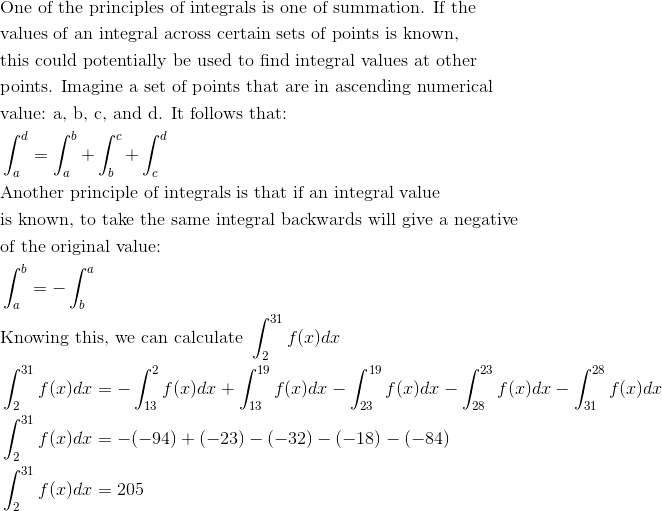### Example Question #4 : Basic Properties Of Definite Integrals (Additivity And Linearity)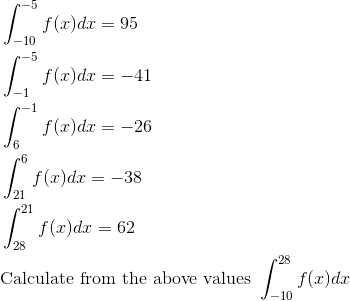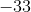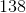Explanation: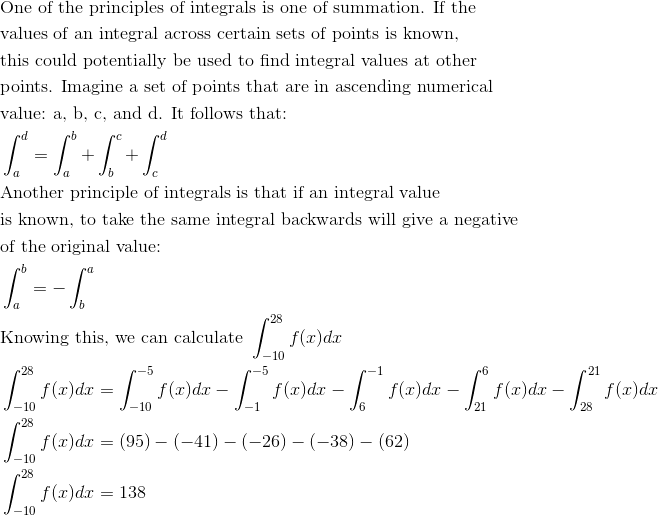### Example Question #5 : Basic Properties Of Definite Integrals (Additivity And Linearity)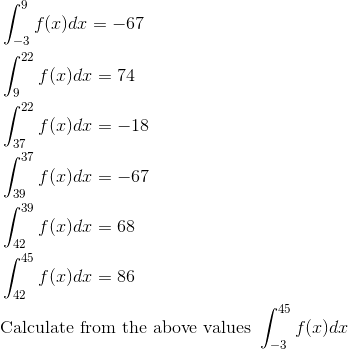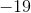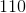Explanation: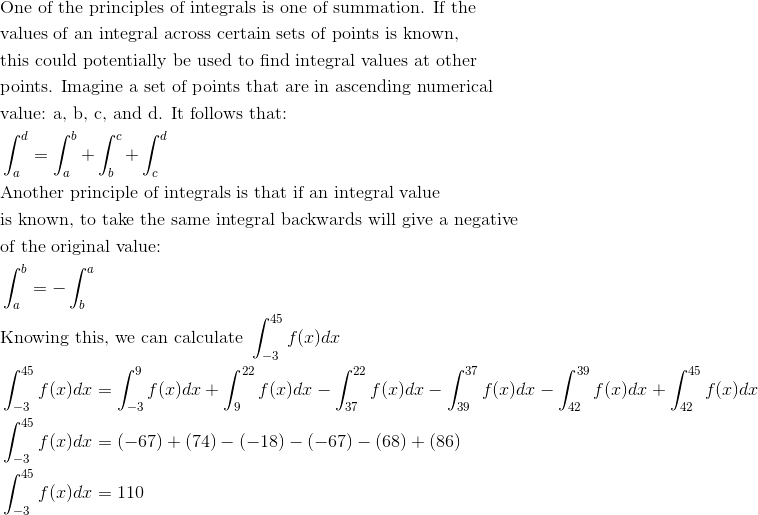### Example Question #1 : Basic Properties Of Definite Integrals (Additivity And Linearity)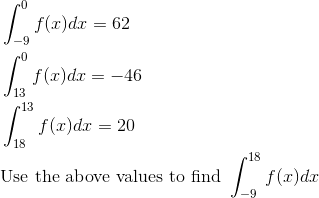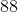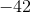Explanation: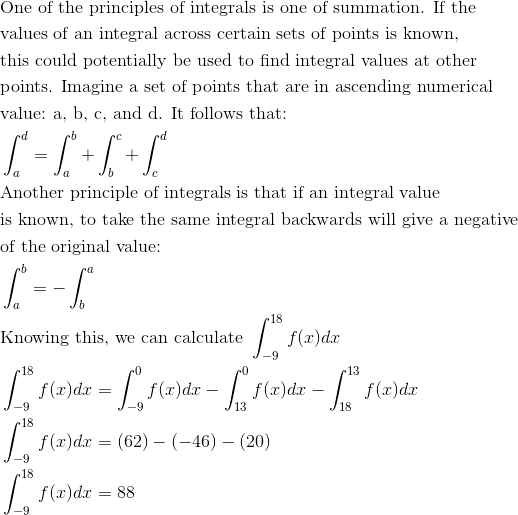### Example Question #7 : Basic Properties Of Definite Integrals (Additivity And Linearity)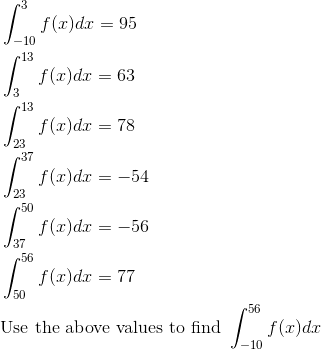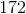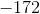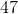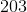Explanation: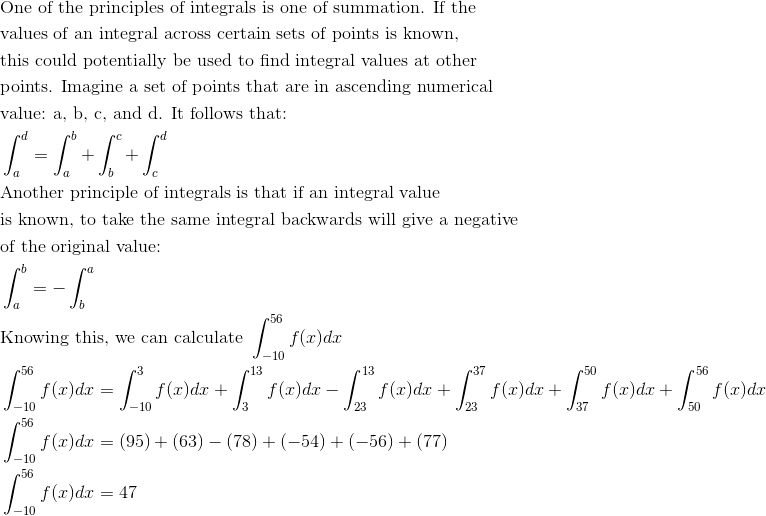### Example Question #8 : Basic Properties Of Definite Integrals (Additivity And Linearity)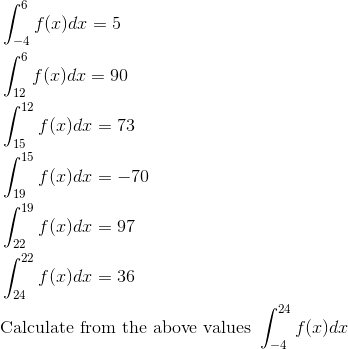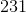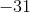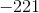Explanation: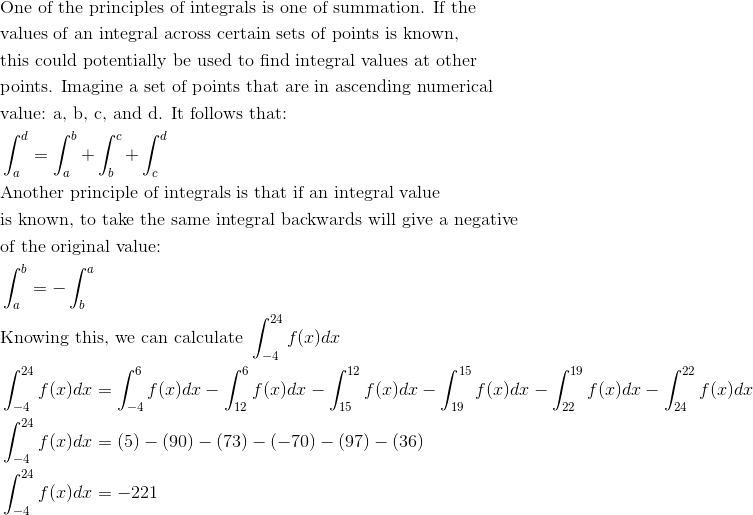### Example Question #9 : Basic Properties Of Definite Integrals (Additivity And Linearity)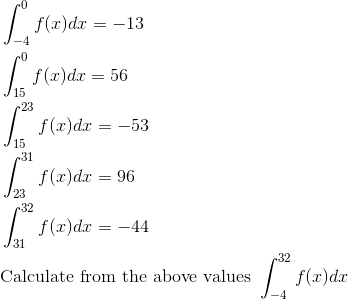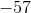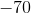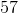Explanation: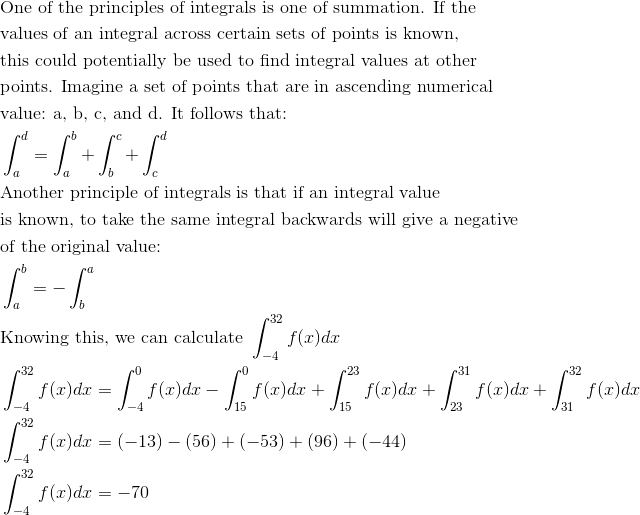### Example Question #10 : Basic Properties Of Definite Integrals (Additivity And Linearity)